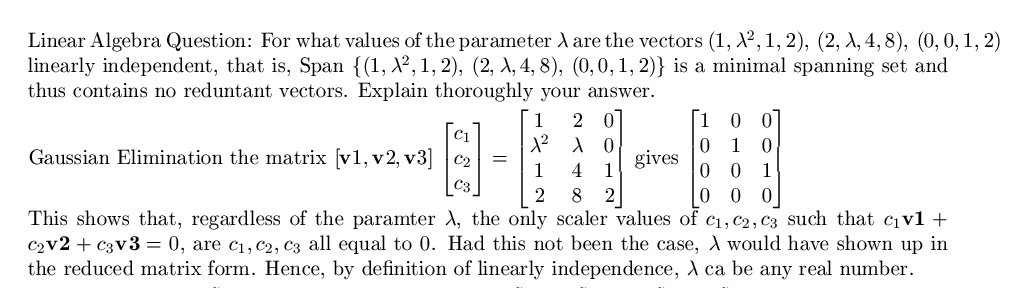# Thread: Linear Algebra: Linear Independence question

1. ## Linear Algebra: Linear Independence question

Hey guys,

Can you tell me if this solution looks alright?Thanks!
James

2. Sorry the picture didn't come up. Here it is again.3. The row reduction looks good to me. I'm not sure I would your matrix equation the way you did. I would write it this way:

$\displaystyle \left[\begin{array}{rrr}\mathbf{v}_{1} &\mathbf{v}_{2} &\mathbf{v}_{3}\end{array}\right]\left[\begin{array}{r}c_{1}\\ c_{2}\\ c_{3}\end{array}\right]=\left[\begin{array}{rrr}1 &2 &0\\ \lambda^{2} &\lambda &0\\ 1 &4 &1\\ 2 &8 &2\end{array}\right]\left[\begin{array}{r}c_{1}\\ c_{2}\\ c_{3}\end{array}\right].$

Your column matrix

$\displaystyle \left[\begin{array}{r}c_{1}\\ c_{2}\\ c_{3}\end{array}\right]$

disappeared on the RHS for some reason. Other than that, as I say, it looks good to me!

[EDIT] See Deveno's post for a correction.

4. one must be extremely careful in accounting for each step of the row reduction.

i found that to eliminate λ from the row-reduced matrix, it was necessary to divide by λ. this suggests that λ = 0 is a special case.

and indeed, when λ = 0, we have 2(1,0,1,2) + 2(0,0,1,2) = (2,0,4,8), so that {(1,0,1,2), (2,0,4,8), (0,0,1,2)} is a linearly dependent set.

thus the statement: "regardless of the parameter λ, the only scalar values of c1, c2, c3 such that c1v1 + c2v2 + c3v3 = 0 are c1,c2,c3 all 0" is UNTRUE

i have exhibited a counter-example.

#### Search Tags

algebra, independence, linear, question